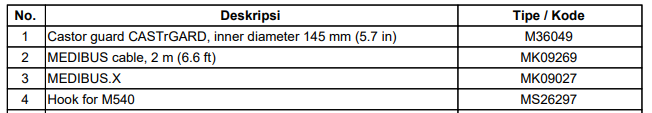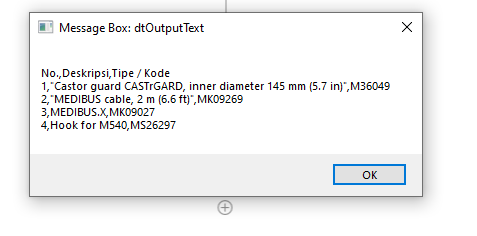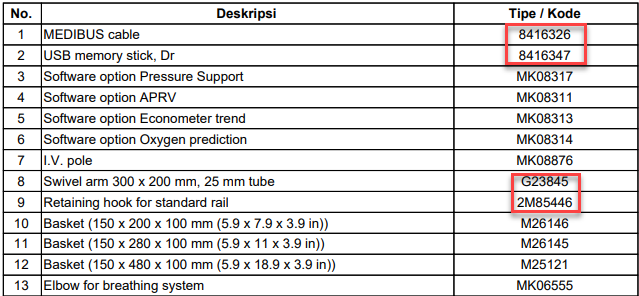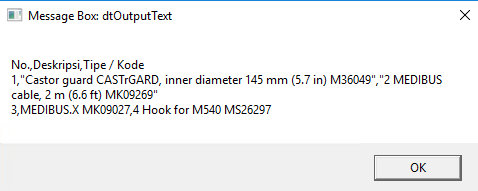# Read Table from PDF and Regex

Hi,
I want to extract the table from pdf like below.I used read pdf text and it was unstructured like this.
"No. Deskripsi Tipe / Kode
1 Castor guard CASTrGARD, inner diameter 145 mm (5.7 in) M36049
2 MEDIBUS cable, 2 m (6.6 ft) MK09269
3 MEDIBUS.X MK09027
4 Hook for M540 MS26297"

Any ways or regex to split it?

This expression might do the trick.

`(?'No'\d+)\s(?'Deskripsi'.*)\s(?'Kode'[M].*)`

1 Like

Check this below workflow attached and I have used your input text which you mentioned above,

Regex:-
`^([0-9]+)\s+(.*)(?=M)([A-z0-9]+)`

Output:-Hope this might help you3 Likes

First, use the “Matches” activity to extract the lines of text that contain the information you want to split. Set the “Input” property to the original text and set the “Pattern” property to “\d+\s+[A-Za-z\s.,()]+\s+[A-Z]+\d+” to match the lines that contain the information you want to split.

Next, use a “For Each” activity to loop through the matches returned by the “Matches” activity. Set the “TypeArgument” property to “System.Text.RegularExpressions.Match”.

Inside the “For Each” loop, use the “Assign” activity to extract the values for each column and add a new DataRow to the DataTable. Here’s an example of how you can extract the values using regular expressions and add a new DataRow:

``````Assign No = System.Text.RegularExpressions.Regex.Match(match.Value, "^\d+").Value
Assign Deskripsi = System.Text.RegularExpressions.Regex.Match(match.Value, "(?<=^\d+\s)[A-Za-z\s.,()]+(?=\s[A-Z]+\d+)").Value
Assign TipeKode = System.Text.RegularExpressions.Regex.Match(match.Value, "(?<=[A-Za-z\s.,()]+\s)[A-Z]+\d+").Value
Assign Tipe = System.Text.RegularExpressions.Regex.Match(TipeKode, "^[A-Z]+").Value
Assign Kode = System.Text.RegularExpressions.Regex.Match(TipeKode, "\d+\$").Value

``````

Finally, use a “Write Range” activity to write the DataTable to a file or Excel sheet.

Hi @Manish540 ,

thanks a lot for the solution, how about the “kode” has different alphabet at the front and sometimes it starts with number instead of alphabet?Appreciate the kind helpHi @moosh

thanks a lot for the solution, how about the “kode” has different alphabet at the front and sometimes it starts with number instead of alphabet?Appreciate the kind helpThanks for the help. Will it work if the “No” is 1-3 digits? and how about if the “Kode” starts with other characters like below.Appreciate the kind helpYou could try this one. Should work for letters/numbers in that last column

`^(?'No'\d+)\s(?'Deskripsi'.*)\s(?'Kode'.*\$)`

1 Like

Thanks a lot. However, it shows number 1 and 2 with no enter break in between.or maybe any idea n how to extract only “Deskripsi” then the “Kode”?